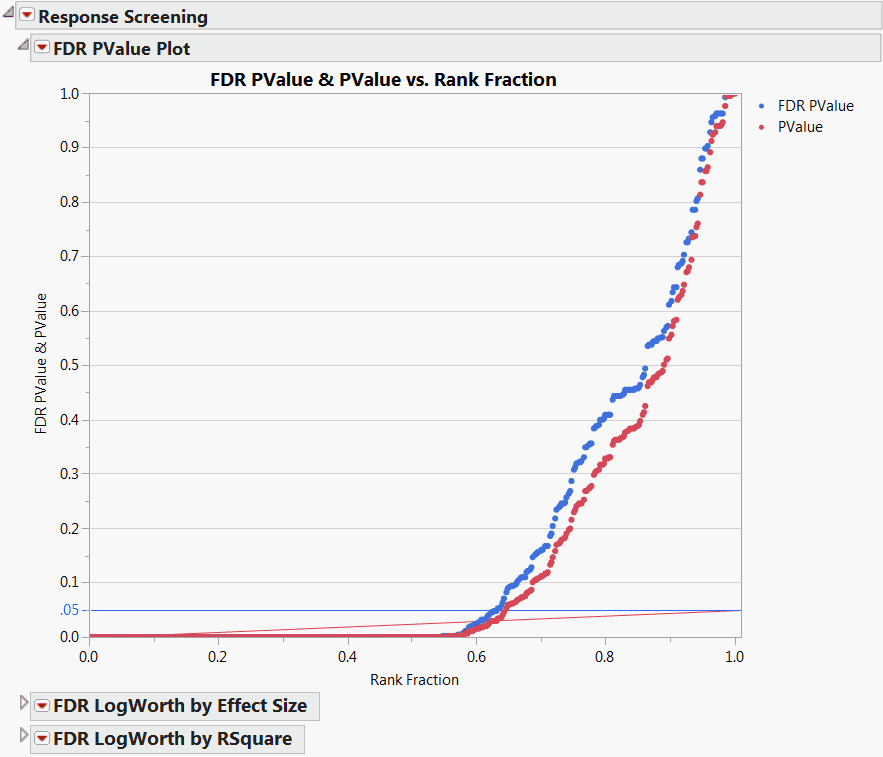Publication date: 08/13/2020

## FDR PValue Plot

The FDR PValue Plot report shows a plot of FDR PValues and PValues against the Rank Fraction. The Rank Fraction ranks the PValues in order of decreasing significance. FDR PValues are plotted in blue and PValues in red.

A blue horizontal line shows the 0.05 significance level. Note that you can change this level by double-clicking the vertical axis, removing the current reference line, and adding a new reference line.

A red increasing line provides an FDR threshold for unadjusted p-values. A p-value falls below the red line precisely when the FDR-adjusted p-value falls below the blue line. This enables you to read significance relative to the FDR from either the adjusted or unadjusted p-values.

Figure 21.5 shows the FDR PValue Plot for the Probe.jmp sample data table. Note that some tests are significant according to the usual p-value but not according to the FDR p-value.

Figure 21.5 FDR PValue Plot